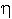# 3 Description Directory for HD-72 Datum and Reference System

Name of the Datum: HD-72, Hungarian Datum of 1972.

Description:
The HD-72 is the Hungarian Geodetic Reference System located and oriented relatively at the Geodetic Datum point Szőlőhegy. It is defined in the procedure of adjustment of the Hungarian Astrogeodetic Network, and on the IUGG GRS 1967 reference ellipsoid under condition of () = minimum, as written for the deflections of vertical at the Laplace-points.
Date of introduction: 1972-1975

Reference ellipsoid:
a) Name: IUGG GRS 1967
b) Axes: a = 6 378 160 m and b = 6 356 774.516 m
Datum station:
a) Name: Szőlőhegy, Geodetic Datum point.
b) Coordinates on reference ellipsoid:= 47°17´ 32.6156"= 19°36´ 09.9865"
h = H + N = 229.24 m + 6.56 m = 235.80 m

where h = height above the ellipsoid, H = height above the Baltic sea, N = geoid undulation

or in rectangular coordinates:
X = 4 082 838.04 m
Y = 1 454 055.97 m
Z = 4 664 062.68 m

Ellipsoidal azimuth from Szőlőhegy to Erdőhegy:= 209°55´ 26.64"
c) Astronomical coordinates:Ast = 47°17'30.44"Ast = 19°36'10.18"
Astronomical azimuth from Szőlőhegy to Erdőhegy:Ast = 209°55´ 27.79"
Origin of altitude:
Fundamental bench mark Nadap,
H = 173.1638 m above Baltic sea.
Type of altitude:
Normal heights in m (H).
Geoid undulation: N

Deflection of vertical:andSource of information:
Joó, I., 1974; Joó, I. et al., 1985; Czobor, Á., 1993; Personal communications (see Chapter 8).Back to the Description directory of the Hungarian Geodetic ReferenceJump to the Homepage of Department of Cartography and Geoinformatics, Eötvös University, Budapest